OpenCV  4.1.2 Open Source Computer Vision
Porting anisotropic image segmentation on G-API

# Introduction

In this tutorial you will learn:

• How an existing algorithm can be transformed into a G-API computation (graph);
• How to inspect and profile G-API graphs;
• How to customize graph execution without changing its code.

This tutorial is based on Anisotropic image segmentation by a gradient structure tensor.

# Quick start: using OpenCV backend

Before we start, let's review the original algorithm implementation:

#include <iostream>
using namespace cv;
using namespace std;
void calcGST(const Mat& inputImg, Mat& imgCoherencyOut, Mat& imgOrientationOut, int w);
int main()
{
int W = 52; // window size is WxW
double C_Thr = 0.43; // threshold for coherency
int LowThr = 35; // threshold1 for orientation, it ranges from 0 to 180
int HighThr = 57; // threshold2 for orientation, it ranges from 0 to 180
if (imgIn.empty()) //check whether the image is loaded or not
{
cout << "ERROR : Image cannot be loaded..!!" << endl;
return -1;
}
Mat imgCoherency, imgOrientation;
calcGST(imgIn, imgCoherency, imgOrientation, W);
Mat imgCoherencyBin;
imgCoherencyBin = imgCoherency > C_Thr;
Mat imgOrientationBin;
inRange(imgOrientation, Scalar(LowThr), Scalar(HighThr), imgOrientationBin);
Mat imgBin;
imgBin = imgCoherencyBin & imgOrientationBin;
normalize(imgCoherency, imgCoherency, 0, 255, NORM_MINMAX);
normalize(imgOrientation, imgOrientation, 0, 255, NORM_MINMAX);
imwrite("result.jpg", 0.5*(imgIn + imgBin));
imwrite("Coherency.jpg", imgCoherency);
imwrite("Orientation.jpg", imgOrientation);
return 0;
}
void calcGST(const Mat& inputImg, Mat& imgCoherencyOut, Mat& imgOrientationOut, int w)
{
Mat img;
inputImg.convertTo(img, CV_32F);
// GST components calculation (start)
// J = (J11 J12; J12 J22) - GST
Mat imgDiffX, imgDiffY, imgDiffXY;
Sobel(img, imgDiffX, CV_32F, 1, 0, 3);
Sobel(img, imgDiffY, CV_32F, 0, 1, 3);
multiply(imgDiffX, imgDiffY, imgDiffXY);
Mat imgDiffXX, imgDiffYY;
multiply(imgDiffX, imgDiffX, imgDiffXX);
multiply(imgDiffY, imgDiffY, imgDiffYY);
Mat J11, J22, J12; // J11, J22 and J12 are GST components
boxFilter(imgDiffXX, J11, CV_32F, Size(w, w));
boxFilter(imgDiffYY, J22, CV_32F, Size(w, w));
boxFilter(imgDiffXY, J12, CV_32F, Size(w, w));
// GST components calculation (stop)
// eigenvalue calculation (start)
// lambda1 = J11 + J22 + sqrt((J11-J22)^2 + 4*J12^2)
// lambda2 = J11 + J22 - sqrt((J11-J22)^2 + 4*J12^2)
Mat tmp1, tmp2, tmp3, tmp4;
tmp1 = J11 + J22;
tmp2 = J11 - J22;
multiply(tmp2, tmp2, tmp2);
multiply(J12, J12, tmp3);
sqrt(tmp2 + 4.0 * tmp3, tmp4);
Mat lambda1, lambda2;
lambda1 = tmp1 + tmp4; // biggest eigenvalue
lambda2 = tmp1 - tmp4; // smallest eigenvalue
// eigenvalue calculation (stop)
// Coherency calculation (start)
// Coherency = (lambda1 - lambda2)/(lambda1 + lambda2)) - measure of anisotropism
// Coherency is anisotropy degree (consistency of local orientation)
divide(lambda1 - lambda2, lambda1 + lambda2, imgCoherencyOut);
// Coherency calculation (stop)
// orientation angle calculation (start)
// tan(2*Alpha) = 2*J12/(J22 - J11)
// Alpha = 0.5 atan2(2*J12/(J22 - J11))
phase(J22 - J11, 2.0*J12, imgOrientationOut, true);
imgOrientationOut = 0.5*imgOrientationOut;
// orientation angle calculation (stop)
}

## Examining calcGST()

The function calcGST() is clearly an image processing pipeline:

Considering the above, calcGST() is a great candidate to start with. In the original code, its prototype is defined like this:

void calcGST(const Mat& inputImg, Mat& imgCoherencyOut, Mat& imgOrientationOut, int w);

With G-API, we can define it as follows:

void calcGST(const cv::GMat& inputImg, cv::GMat& imgCoherencyOut, cv::GMat& imgOrientationOut, int w);

It is important to understand that the new G-API based version of calcGST() will just produce a compute graph, in contrast to its original version, which actually calculates the values. This is a principal difference – G-API based functions like this are used to construct graphs, not to process the actual data.

Let's start implementing calcGST() with calculation of $$J$$ matrix. This is how the original code looks like:

void calcGST(const Mat& inputImg, Mat& imgCoherencyOut, Mat& imgOrientationOut, int w)
{
Mat img;
inputImg.convertTo(img, CV_32F);
// GST components calculation (start)
// J = (J11 J12; J12 J22) - GST
Mat imgDiffX, imgDiffY, imgDiffXY;
Sobel(img, imgDiffX, CV_32F, 1, 0, 3);
Sobel(img, imgDiffY, CV_32F, 0, 1, 3);
multiply(imgDiffX, imgDiffY, imgDiffXY);

Here we need to declare output objects for every new operation (see img as a result for cv::Mat::convertTo, imgDiffX and others as results for cv::Sobel and cv::multiply).

The G-API analogue is listed below:

void calcGST(const cv::GMat& inputImg, cv::GMat& imgCoherencyOut, cv::GMat& imgOrientationOut, int w)
{
auto img = cv::gapi::convertTo(inputImg, CV_32F);
auto imgDiffX = cv::gapi::Sobel(img, CV_32F, 1, 0, 3);
auto imgDiffY = cv::gapi::Sobel(img, CV_32F, 0, 1, 3);
auto imgDiffXY = cv::gapi::mul(imgDiffX, imgDiffY);

This snippet demonstrates the following syntactic difference between G-API and traditional OpenCV:

• All standard G-API functions are by default placed in "cv::gapi" namespace;
• G-API operations return its results – there's no need to pass extra "output" parameters to the functions.

Note – this code is also using auto – types of intermediate objects like img, imgDiffX, and so on are inferred automatically by the C++ compiler. In this example, the types are determined by G-API operation return values which all are cv::GMat.

G-API standard kernels are trying to follow OpenCV API conventions whenever possible – so cv::gapi::sobel takes the same arguments as cv::Sobel, cv::gapi::mul follows cv::multiply, and so on (except having a return value).

The rest of calcGST() function can be implemented the same way trivially. Below is its full source code:

void calcGST(const cv::GMat& inputImg, cv::GMat& imgCoherencyOut, cv::GMat& imgOrientationOut, int w)
{
auto img = cv::gapi::convertTo(inputImg, CV_32F);
auto imgDiffX = cv::gapi::Sobel(img, CV_32F, 1, 0, 3);
auto imgDiffY = cv::gapi::Sobel(img, CV_32F, 0, 1, 3);
auto imgDiffXY = cv::gapi::mul(imgDiffX, imgDiffY);
auto imgDiffXX = cv::gapi::mul(imgDiffX, imgDiffX);
auto imgDiffYY = cv::gapi::mul(imgDiffY, imgDiffY);
auto J11 = cv::gapi::boxFilter(imgDiffXX, CV_32F, cv::Size(w, w));
auto J22 = cv::gapi::boxFilter(imgDiffYY, CV_32F, cv::Size(w, w));
auto J12 = cv::gapi::boxFilter(imgDiffXY, CV_32F, cv::Size(w, w));
auto tmp1 = J11 + J22;
auto tmp2 = J11 - J22;
auto tmp22 = cv::gapi::mul(tmp2, tmp2);
auto tmp3 = cv::gapi::mul(J12, J12);
auto tmp4 = cv::gapi::sqrt(tmp22 + 4.0*tmp3);
auto lambda1 = tmp1 + tmp4;
auto lambda2 = tmp1 - tmp4;
imgCoherencyOut = (lambda1 - lambda2) / (lambda1 + lambda2);
imgOrientationOut = 0.5*cv::gapi::phase(J22 - J11, 2.0*J12, true);
}

## Running G-API graph

After calcGST() is defined in G-API language, we can construct a graph based on it and finally run it – pass input image and obtain result. Before we do it, let's have a look how original code looked like:

Mat imgCoherency, imgOrientation;
calcGST(imgIn, imgCoherency, imgOrientation, W);
Mat imgCoherencyBin;
imgCoherencyBin = imgCoherency > C_Thr;
Mat imgOrientationBin;
inRange(imgOrientation, Scalar(LowThr), Scalar(HighThr), imgOrientationBin);
Mat imgBin;
imgBin = imgCoherencyBin & imgOrientationBin;
normalize(imgCoherency, imgCoherency, 0, 255, NORM_MINMAX);
normalize(imgOrientation, imgOrientation, 0, 255, NORM_MINMAX);
imwrite("result.jpg", 0.5*(imgIn + imgBin));
imwrite("Coherency.jpg", imgCoherency);
imwrite("Orientation.jpg", imgOrientation);

G-API-based functions like calcGST() can't be applied to input data directly, since it is a construction code, not the processing code. In order to run computations, a special object of class cv::GComputation needs to be created. This object wraps our G-API code (which is a composition of G-API data and operations) into a callable object, similar to C++11 std::function<>.

cv::GComputation class has a number of constructors which can be used to define a graph. Generally, user needs to pass graph boundaries – input and output objects, on which a GComputation is defined. Then G-API analyzes the call flow from outputs to inputs and reconstructs the graph with operations in-between the specified boundaries. This may sound complex, however in fact the code looks like this:

// Calculate Gradient Structure Tensor and post-process it for output with G-API
cv::GMat imgCoherency, imgOrientation;
calcGST(in, imgCoherency, imgOrientation, W);
cv::GMat imgCoherencyBin = imgCoherency > C_Thr;
cv::GMat imgOrientationBin = cv::gapi::inRange(imgOrientation, LowThr, HighThr);
cv::GMat imgBin = imgCoherencyBin & imgOrientationBin;
cv::GMat out = cv::gapi::addWeighted(in, 0.5, imgBin, 0.5, 0.0);
// Normalize extra outputs
cv::GMat imgCoherencyNorm = cv::gapi::normalize(imgCoherency, 0, 255, cv::NORM_MINMAX);
cv::GMat imgOrientationNorm = cv::gapi::normalize(imgOrientation, 0, 255, cv::NORM_MINMAX);
// Capture the graph into object segm
cv::GComputation segm(cv::GIn(in), cv::GOut(out, imgCoherencyNorm, imgOrientationNorm));
// Define cv::Mats for output data
cv::Mat imgOut, imgOutCoherency, imgOutOrientation;
// Run the graph
segm.apply(cv::gin(imgIn), cv::gout(imgOut, imgOutCoherency, imgOutOrientation));
cv::imwrite("result.jpg", imgOut);
cv::imwrite("Coherency.jpg", imgOutCoherency);
cv::imwrite("Orientation.jpg", imgOutOrientation);

Note that this code slightly changes from the original one: forming up the resulting image is also a part of the pipeline (done with cv::gapi::addWeighted).

Result of this G-API pipeline bit-exact matches the original one (given the same input image):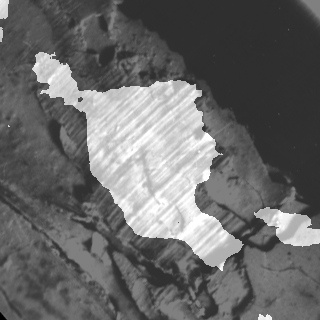Segmentation result with G-API

## G-API initial version: full listing

Below is the full listing of the initial anisotropic image segmentation port on G-API:

#include <iostream>
#include <utility>
#include "opencv2/gapi.hpp"
void calcGST(const cv::GMat& inputImg, cv::GMat& imgCoherencyOut, cv::GMat& imgOrientationOut, int w);
int main()
{
int W = 52; // window size is WxW
double C_Thr = 0.43; // threshold for coherency
int LowThr = 35; // threshold1 for orientation, it ranges from 0 to 180
int HighThr = 57; // threshold2 for orientation, it ranges from 0 to 180
if (imgIn.empty()) //check whether the image is loaded or not
{
std::cout << "ERROR : Image cannot be loaded..!!" << std::endl;
return -1;
}
// Calculate Gradient Structure Tensor and post-process it for output with G-API
cv::GMat imgCoherency, imgOrientation;
calcGST(in, imgCoherency, imgOrientation, W);
cv::GMat imgCoherencyBin = imgCoherency > C_Thr;
cv::GMat imgOrientationBin = cv::gapi::inRange(imgOrientation, LowThr, HighThr);
cv::GMat imgBin = imgCoherencyBin & imgOrientationBin;
cv::GMat out = cv::gapi::addWeighted(in, 0.5, imgBin, 0.5, 0.0);
// Normalize extra outputs
cv::GMat imgCoherencyNorm = cv::gapi::normalize(imgCoherency, 0, 255, cv::NORM_MINMAX);
cv::GMat imgOrientationNorm = cv::gapi::normalize(imgOrientation, 0, 255, cv::NORM_MINMAX);
// Capture the graph into object segm
cv::GComputation segm(cv::GIn(in), cv::GOut(out, imgCoherencyNorm, imgOrientationNorm));
// Define cv::Mats for output data
cv::Mat imgOut, imgOutCoherency, imgOutOrientation;
// Run the graph
segm.apply(cv::gin(imgIn), cv::gout(imgOut, imgOutCoherency, imgOutOrientation));
cv::imwrite("result.jpg", imgOut);
cv::imwrite("Coherency.jpg", imgOutCoherency);
cv::imwrite("Orientation.jpg", imgOutOrientation);
return 0;
}
void calcGST(const cv::GMat& inputImg, cv::GMat& imgCoherencyOut, cv::GMat& imgOrientationOut, int w)
{
auto img = cv::gapi::convertTo(inputImg, CV_32F);
auto imgDiffX = cv::gapi::Sobel(img, CV_32F, 1, 0, 3);
auto imgDiffY = cv::gapi::Sobel(img, CV_32F, 0, 1, 3);
auto imgDiffXY = cv::gapi::mul(imgDiffX, imgDiffY);
auto imgDiffXX = cv::gapi::mul(imgDiffX, imgDiffX);
auto imgDiffYY = cv::gapi::mul(imgDiffY, imgDiffY);
auto J11 = cv::gapi::boxFilter(imgDiffXX, CV_32F, cv::Size(w, w));
auto J22 = cv::gapi::boxFilter(imgDiffYY, CV_32F, cv::Size(w, w));
auto J12 = cv::gapi::boxFilter(imgDiffXY, CV_32F, cv::Size(w, w));
auto tmp1 = J11 + J22;
auto tmp2 = J11 - J22;
auto tmp22 = cv::gapi::mul(tmp2, tmp2);
auto tmp3 = cv::gapi::mul(J12, J12);
auto tmp4 = cv::gapi::sqrt(tmp22 + 4.0*tmp3);
auto lambda1 = tmp1 + tmp4;
auto lambda2 = tmp1 - tmp4;
imgCoherencyOut = (lambda1 - lambda2) / (lambda1 + lambda2);
imgOrientationOut = 0.5*cv::gapi::phase(J22 - J11, 2.0*J12, true);
}

# Inspecting the initial version

After we have got the initial working version of our algorithm working with G-API, we can use it to inspect and learn how G-API works. This chapter covers two aspects: understanding the graph structure, and memory profiling.

## Understanding the graph structure

G-API stands for "Graph API", but did you mention any graphs in the above example? It was one of the initial design goals – G-API was designed with expressions in mind to make adoption and porting process more straightforward. People usually don't think in terms of Nodes and Edges when writing ordinary code, and so G-API, while being a Graph API, doesn't force its users to do that.

However, a graph is still built implicitly when a cv::GComputation object is defined. It may be useful to inspect how the resulting graph looks like to check if it is generated correctly and if it really represents our alrogithm. It is also useful to learn the structure of the graph to see if it has any redundancies.

G-API allows to dump generated graphs to .dot files which then could be visualized with Graphviz, a popular open graph visualization software.

In order to dump our graph to a .dot file, set GRAPH_DUMP_PATH to a file name before running the application, e.g.:

$GRAPH_DUMP_PATH=segm.dot ./bin/example_tutorial_porting_anisotropic_image_segmentation_gapi  Now this file can be visalized with a dot command like this: $ dot segm.dot -Tpng -o segm.png


or viewed instantly with xdot command (please refer to your distribution/operating system documentation on how to install these packages).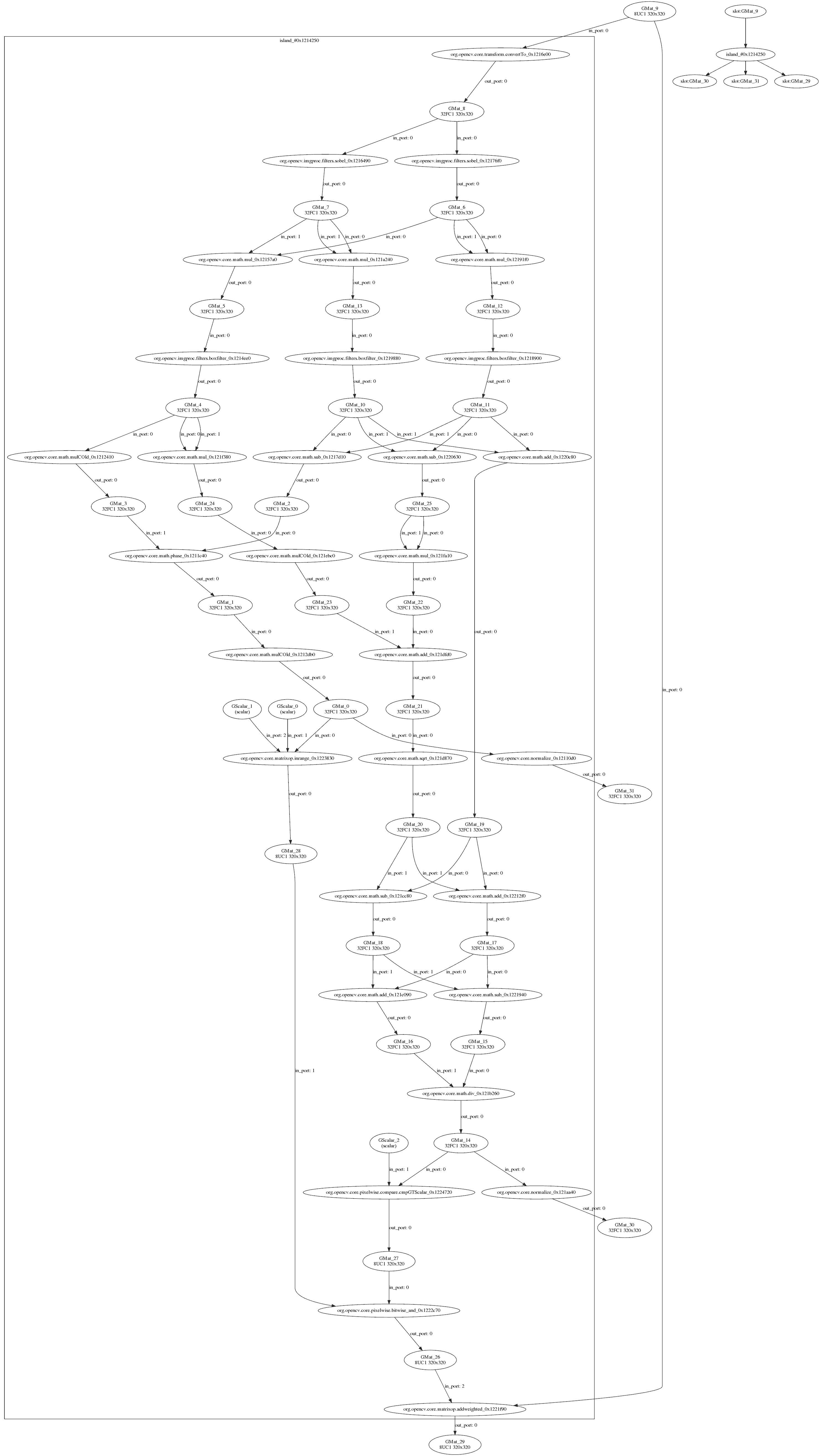Anisotropic image segmentation graph

The above diagram demonstrates a number of interesting aspects of G-API's internal algorithm representation:

1. G-API underlying graph is a bipartite graph: it consists of Operation and Data nodes such that a Data node can only be connected to an Operation node, Operation node can only be connected to a Data node, and nodes of a single kind are never connected directly.
2. Graph is directed - every edge in the graph has a direction.
3. Graph "begins" and "ends" with a Data kind of nodes.
4. A Data node can have only a single writer and multiple readers.
5. An Operation node may have multiple inputs, though every input must have an unique port number (among inputs).
6. An Operation node may have multiple outputs, and every output must have an unique port number (among outputs).

## Measuring memory footprint

Let's measure and compare memory footprint of the algorithm in its two versions: G-API-based and OpenCV-based. At the moment, G-API version is also OpenCV-based since it fallbacks to OpenCV functions inside.

On GNU/Linux, application memory footprint can be profiled with Valgrind. On Debian/Ubuntu systems it can be installed like this (assuming you have administrator privileges):

$sudo apt-get install valgrind massif-visualizer  Once installed, we can collect memory profiles easily for our two algorithm versions: $ valgrind --tool=massif --massif-out-file=ocv.out ./bin/example_tutorial_anisotropic_image_segmentation
==6101== Massif, a heap profiler
==6101== Copyright (C) 2003-2015, and GNU GPL'd, by Nicholas Nethercote
==6101== Using Valgrind-3.11.0 and LibVEX; rerun with -h for copyright info
==6101== Command: ./bin/example_tutorial_anisotropic_image_segmentation
==6101==
==6101==
$valgrind --tool=massif --massif-out-file=gapi.out ./bin/example_tutorial_porting_anisotropic_image_segmentation_gapi ==6117== Massif, a heap profiler ==6117== Copyright (C) 2003-2015, and GNU GPL'd, by Nicholas Nethercote ==6117== Using Valgrind-3.11.0 and LibVEX; rerun with -h for copyright info ==6117== Command: ./bin/example_tutorial_porting_anisotropic_image_segmentation_gapi ==6117== ==6117==  Once done, we can inspect the collected profiles with Massif Visualizer (installed in the above step). Below is the visualized memory profile of the original OpenCV version of the algorithm: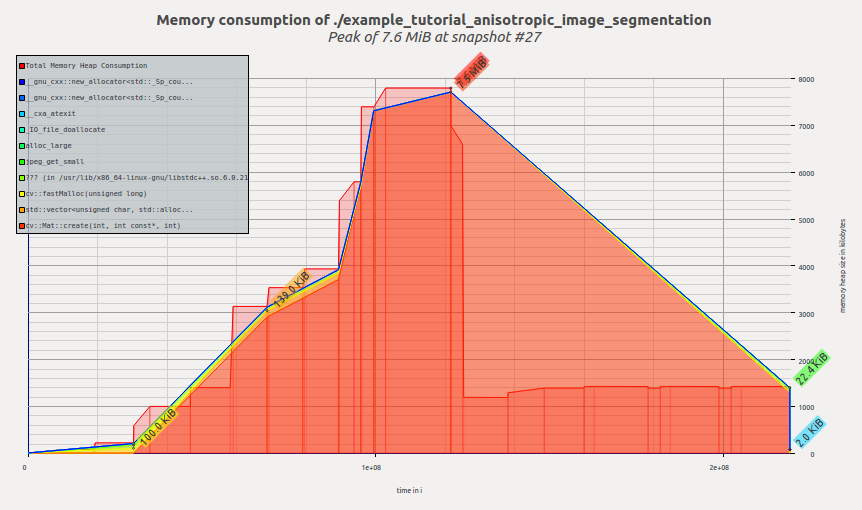Memory profile: original Anisotropic Image Segmentation sample We see that memory is allocated as the application executes, reaching its peak in the calcGST() function; then the footprint drops as calcGST() completes its execution and all temporary buffers are freed. Massif reports us peak memory consumption of 7.6 MiB. Now let's have a look on the profile of G-API version: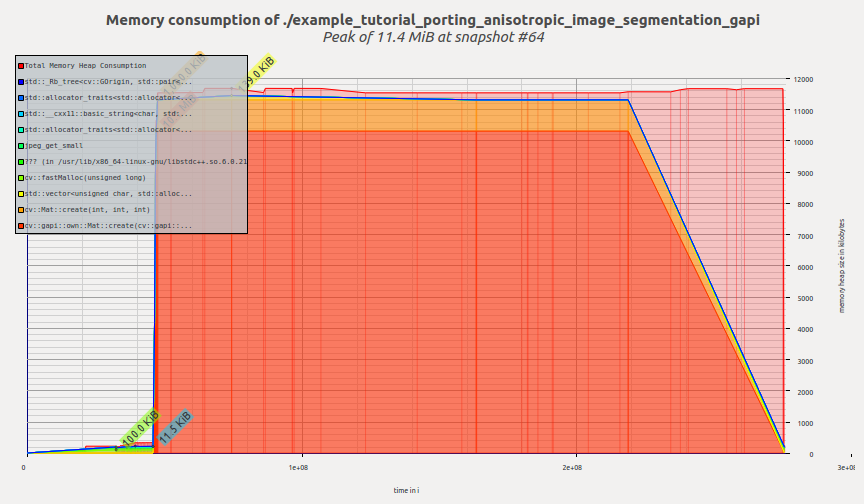Memory profile: G-API port of Anisotropic Image Segmentation sample Once G-API computation is created and its execution starts, G-API allocates all required memory at once and then the memory profile remains flat until the termination of the program. Massif reports us peak memory consumption of 11.4 MiB. A reader may ask a right question at this point – is G-API that bad? What is the reason in using it than? Hopefully, it is not. The reason why we see here an increased memory consumption is because the default naive OpenCV-based backend is used to execute this graph. This backend serves mostly for quick prototyping and debugging algorithms before offload/further optimization. This backend doesn't utilize any complex memory management strategies yet since it is not its point at the moment. In the following chapter, we'll learn about Fluid backend and see how the same G-API code can run in a completely different model (and the footprint shrunk to a number of kilobytes). # Backends and kernels This chapter covers how a G-API computation can be executed in a special way – e.g. offloaded to another device, or scheduled with a special intelligence. G-API is designed to make its graphs portable – it means that once a graph is defined in G-API terms, no changes should be required in it if we want to run it on CPU or on GPU or on both devices at once. G-API High-level overview and G-API Kernel API shed more light on technical details which make it possible. In this chapter, we will utilize G-API Fluid backend to make our graph cache-efficient on CPU. G-API defines backend as the lower-level entity which knows how to run kernels. Backends may have (and, in fact, do have) different Kernel APIs which are used to program and integrate kernels for that backends. In this context, kernel is an implementation of an operation, which is defined on the top API level (see G_TYPED_KERNEL() macro). Backend is a thing which is aware of device & platform specifics, and which executes its kernels with keeping that specifics in mind. For example, there may be Halide backend which allows to write (implement) G-API operations in Halide language and then generate functional Halide code for portions of G-API graph which map well there. ## Running a graph with a Fluid backend OpenCV 4.0 is bundled with two G-API backends – the default "OpenCV" which we just used, and a special "Fluid" backend. Fluid backend reorganizes the execution to save memory and to achieve near-perfect cache locality, implementing so-called "streaming" model of execution. In order to start using Fluid kernels, we need first to include appropriate header files (which are not included by default): #include "opencv2/gapi/fluid/core.hpp" // Fluid Core kernel library #include "opencv2/gapi/fluid/imgproc.hpp" // Fluid ImgProc kernel library Once these headers are included, we can form up a new kernel package and specify it to G-API: // Prepare the kernel package and run the graph cv::gapi::GKernelPackage fluid_kernels = cv::gapi::combine // Define a custom kernel package: (cv::gapi::core::fluid::kernels(), // ...with Fluid Core kernels cv::gapi::imgproc::fluid::kernels()); // ...and Fluid ImgProc kernels In G-API, kernels (or operation implementations) are objects. Kernels are organized into collections, or kernel packages, represented by class cv::gapi::GKernelPackage. The main purpose of a kernel package is to capture which kernels we would like to use in our graph, and pass it as a graph compilation option: segm.apply(cv::gin(imgIn), // Input data vector cv::gout(imgOut, imgOutCoherency, imgOutOrientation), // Output data vector cv::compile_args(fluid_kernels)); // Kernel package to use Traditional OpenCV is logically divided into modules, with every module providing a set of functions. In G-API, there are also "modules" which are represented as kernel packages provided by a particular backend. In this example, we pass Fluid kernel packages to G-API to utilize appropriate Fluid functions in our graph. Kernel packages are combinable – in the above example, we take "Core" and "ImgProc" Fluid kernel packages and combine it into a single one. See documentation reference on cv::gapi::combine. If no kernel packages are specified in options, G-API is using default package which consists of default OpenCV implementations and thus G-API graphs are executed via OpenCV functions by default. OpenCV backend provides broader functional coverage than any other backend. If a kernel package is specified, like in this example, then it is being combined with the default. It means that user-specified implementations will replace default implementations in case of conflict. ## Troubleshooting and customization After the above modifications, (in OpenCV 4.0) the app should crash with a message like this:$ ./bin/example_tutorial_porting_anisotropic_image_segmentation_gapi_fluid
terminate called after throwing an instance of 'std::logic_error'
what(): .../modules/gapi/src/backends/fluid/gfluidimgproc.cpp:436: Assertion kernelSize.width == 3 && kernelSize.height == 3 in function run failed
Aborted (core dumped)

Fluid backend has a number of limitations in OpenCV 4.0 (see this wiki page for a more up-to-date status). In particular, the Box filter used in this sample supports only static 3x3 kernel size.

We can overcome this problem easily by avoiding G-API using Fluid version of Box filter kernel in this sample. It can be done by removing the appropriate kernel from the kernel package we've just created:

fluid_kernels.remove<cv::gapi::imgproc::GBoxFilter>(); // Remove Fluid Box filter as unsuitable,
// G-API will fall-back to OpenCV there.

Now this kernel package doesn't have any implementation of Box filter kernel interface (specified as a template parameter). As described above, G-API will fall-back to OpenCV to run this kernel now. The resulting code with this change now looks like:

// Prepare the kernel package and run the graph
cv::gapi::GKernelPackage fluid_kernels = cv::gapi::combine // Define a custom kernel package:
(cv::gapi::core::fluid::kernels(), // ...with Fluid Core kernels
cv::gapi::imgproc::fluid::kernels()); // ...and Fluid ImgProc kernels
fluid_kernels.remove<cv::gapi::imgproc::GBoxFilter>(); // Remove Fluid Box filter as unsuitable,
// G-API will fall-back to OpenCV there.
segm.apply(cv::gin(imgIn), // Input data vector
cv::gout(imgOut, imgOutCoherency, imgOutOrientation), // Output data vector
cv::compile_args(fluid_kernels)); // Kernel package to use

Let's examine the memory profile for this sample after we switched to Fluid backend. Now it looks like this: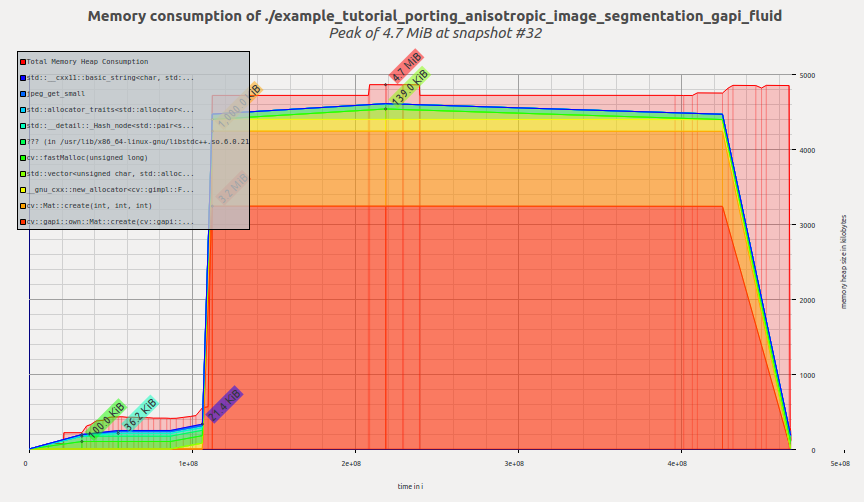Memory profile: G-API/Fluid port of Anisotropic Image Segmentation sample

Now the tool reports 4.7MiB – and we just changed a few lines in our code, without modifying the graph itself! It is a ~2.4X improvement of the previous G-API result, and ~1.6X improvement of the original OpenCV version.

Let's also examine how the internal representation of the graph now looks like. Dumping the graph into .dot would result into a visualization like this: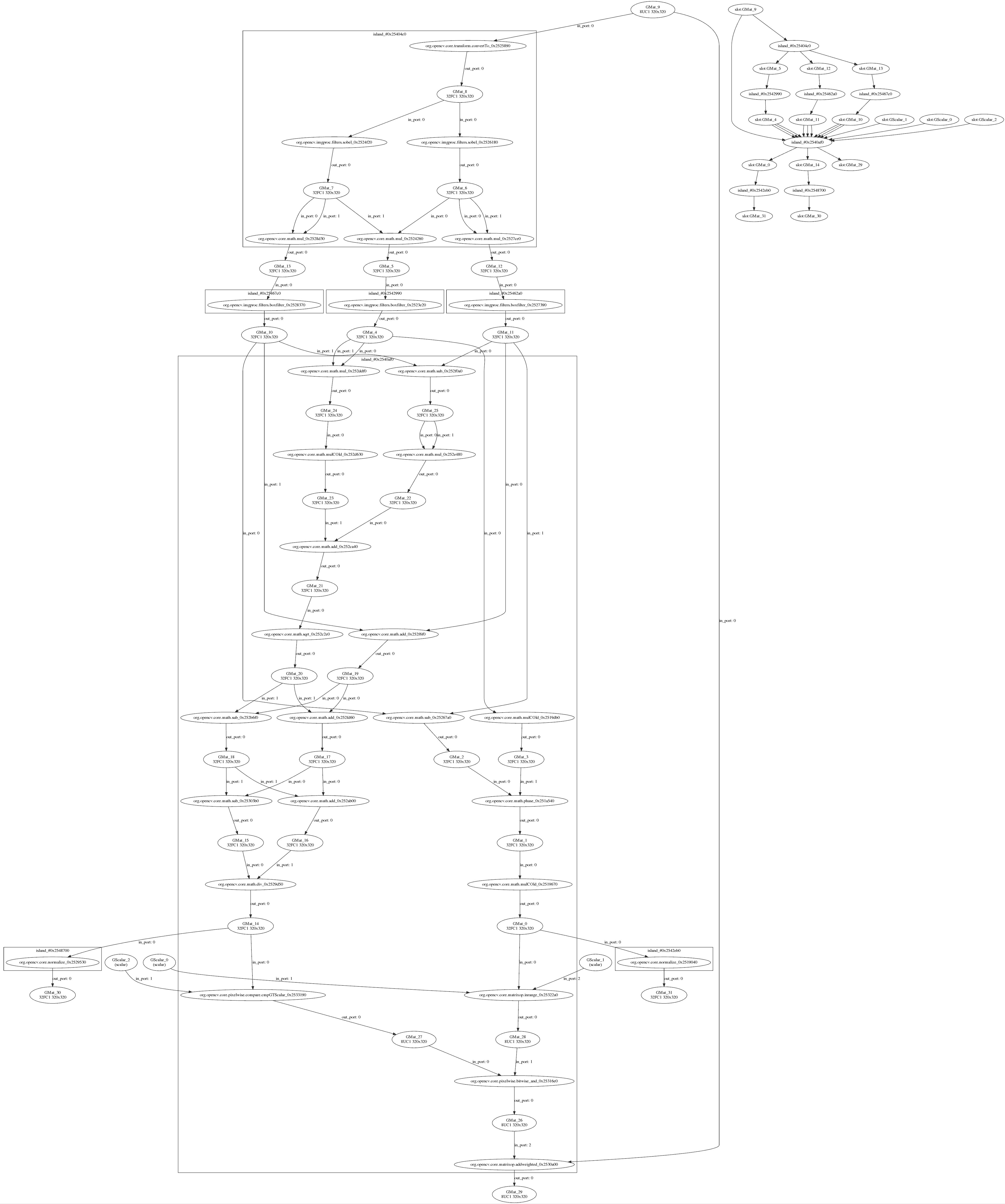Anisotropic image segmentation graph with OpenCV & Fluid kernels

This graph doesn't differ structually from its previous version (in terms of operations and data objects), though a changed layout (on the left side of the dump) is easily noticeable.

The visualization reflects how G-API deals with mixed graphs, also called heterogeneous graphs. The majority of operations in this graph are implemented with Fluid backend, but Box filters are executed by the OpenCV backend. One can easily see that the graph is partitioned (with rectangles). G-API groups connected operations based on their affinity, forming subgraphs (or islands in G-API terminology), and our top-level graph becomes a composition of multiple smaller subgraphs. Every backend determines how its subgraph (island) is executed, so Fluid backend optimizes out memory where possible, and six intermediate buffers accessed by OpenCV Box filters are allocated fully and can't be optimized out.

# Conclusion

This tutorial demonstrates what G-API is and what its key design concepts are, how an algorithm can be ported to G-API, and how to utilize graph model benefits after that.

In OpenCV 4.0, G-API is still in its inception stage – it is more a foundation for all future work, though ready for use even now.

Further, this tutorial will be extended with new chapters on custom kernels programming, parallelism, and more.